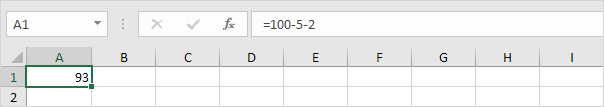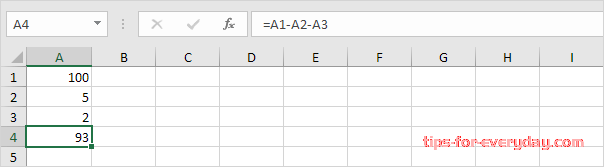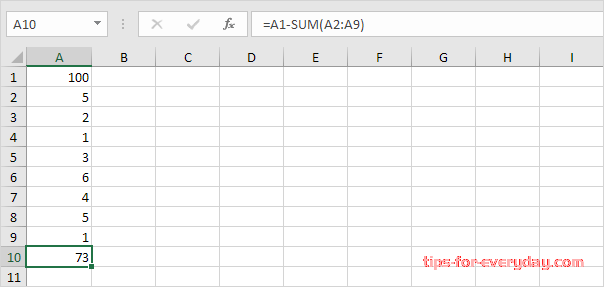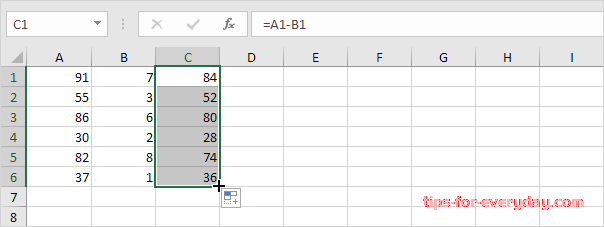Home Excel Content

# 4 ways to Subtract in Excel

Tips 2022-05-19 15:05:07 324

There's no SUBTRACT function in Excel. However, there are several ways to subtract numbers in Excel.

1.

For example, the formula below subtracts numbers in a cell. Simply use the minus sign (-) as the subtraction operator. Don't forget, always start a formula with an equal sign (=).2.

The formula below subtracts the value in cell A2 and the value in cell A3 from the value in cell A1.3.

As you can imagine, this formula can get quite long. Simply use the SUM function to shorten your formula. For example, the formula below subtracts the values in the range A2:A9 from the value in cell A1.4.

To subtract the numbers in column B from the numbers in column A, execute the following steps.

First, subtract the value in cell B1 from the value in cell A1.

Next, select cell C1, click on the lower right corner of cell C1 and drag it down to cell C6.